Score High in Credibility, Accuracy, Practical Value
Solution Matrix Ltd®

# Explaining Fixed vs. Variable Annuities, Example CalculationsWhen parents buy annuities to provide long-term income for their children, the parents become annuity contract holders, and the children become annuitants.

## What Is An Annuity?

Strictly speaking, an annuity is simply a series of cash inflows or outflows, for which a contract agreement governs payment timing and amounts. By this definition, a mortgage loan contract with specified monthly payments may be called an annuity, and a bond purchase that pays interest to the bondholder semiannually is also one. This article defines and illustrates the term annuity, kinds of annuities, and associated calculations, in the context of related terms.

As the term annuity is usually used and understood, however, it refers to a class of financial service products designed to deliver an income stream. Annuities of this kind are created and issued by insurance companies. Buyers may purchase annuities from the issuing insurance company (the issuer), but they can also purchase them through distributors—typically banks or brokerage houses. In any case, the person buying one is the contract holder, and a person receiving payments (a beneficiary) is an annuitant.

Contract holder and receiver may be the same person as when, for instance, people buy them early in life to provide themselves with retirement income later. Or, the contract holder may purchase one to provide income for a child, widow, widower, or someone else.

Annuity issuers normally earn income for the annuitant (and themselves) by using funds paid in by the contract holder as investment principal, to be re-invested in stock shares, bonds, or other securities.

### The Purpose of Annuity Contracts

The annuity contracts describe the life of an annuity, or its duration, the time over which the contract holder pays in, and the time over which the annuitant receives income payments. The stipulated time between these payments is the period.  Contracts normally specify monthly, quarterly, semiannual, or annual periods.

Note that earnings may be retained in the investment account so to add to the investment principal and produce additional earnings of their own, while actual fund withdrawal by the annuitant may occur later.

Annuity contracts also specify conditions for surrender of the investment during its life, that is, conditions under which the contract holder may withdraw all or part of the original investment and cancel the annuity. Surrender typically brings additional fees to the contract holder, but these are usually set by a fee schedule that decreases surrender costs as the end of annuity life approaches.

### Explaining Annuity in Context

Sections below further explains and illustrates annuity in context with related business concepts from the asset management, budgeting, and cost accounting, emphasizing four themes:

• First, defining terms and their releatinships to each other, cindluing Annuity, Annuity Contract, Annutant, and Annuity Life.
• Two, different kinds of annuities available, including fixed and variable payment annuities, single and multiple pay in annuities.
• Third, annuity calculations and formulas, using (1) Ordinary algebraic notation, and (2) Annuity Notation.

## How Do Annuities Serve as Investments?Are Annuities Free of Investment Risk?

Investors and services professionals regard annuities as described above as financial investments because the potential buyer can anticipate the amounts and timings of cash outflows and cash inflow. Analysts can evaluate and compare potential annuity investments using the same cash flow metrics that apply to a broad range of financial investments—metrics such as future value (FV), internal rate of return (IRR), simple return on investment (ROI), effective annual yield, and annual percentage rate (APR).

Most annuities have another characteristic in common with other kinds of investments, namely an element of risk. The value of expected income may be less than certain for several reasons.

• Annuity income depends on the issuers ability to maintain a healthy business and meet its financial obligations. These investments, however, typically lack the kind of government guarantees that often stand behind other investments such as bank savings account deposits, so that issuer failure probably means investment failure as well.
• Another risk comes with so-called variable annuities, where income payments to the beneficiary depend on the issuers ability to bring a good return from its investments. That is, in fact, the reason that income from these investments varies from period to period.
• In contrast to variable instruments, most fixed investments allow the contract holder to "lock in" a rate of return for the recipient. However, if the investor purchases a fixed investment when inflation and interest rates are relatively low, income will also be lower than if the buyer purchases the same investment when inflation and interest rates are higher. That is, when rates rise after the purchase, the annuitant may be locked into lower returns than would now be available from other investments.
• The beneficiary may die before receiving all contracted income.

The purchase contract may include various clauses meant to mitigate such risks, such as an indexing clause that adjust return rates as securities market prices (and inflation and interest rates) change, or a death-benefit clause that transfers income payments to the annuitants estate or beneficiaries in case that person dies before annuity end. However, as with risk-reward situations generally, the lower risk provided by such clauses comes at a cost, in the form of lower returns than comparable investments without them.

## What Kinds of Annuities Are on the Market?What Are Different Kinds of Annuity Payouts?

The potential buyer must select from an extensive set of annuity kinds and classes, where each product has a unique list of features and characteristics. Just a few of the many possibilities appear in examples below.

### What are the differences between fixed and variable (payout) annuity payments?

Annuities are classified first as either fixed or variable, referring to the periodic payments beneficiaries receive:

• For the fixed kinds, each income payment to the annuitant is the same, period to period.
• For the variable kind, income earned can vary from period to period.

Fixed annuities normally pay at a fixed, guaranteed rate. For these, the issuer usually invests the contract holders principal in low-risk government bonds, high-grade corporate stock or bonds, or other relatively "safe" securities. While such investments are low-risk in their own right, the buyer should remember that annuity income will depend on the issuers ability to make payments (i.e., the issuing insurance company's ability to service its claims).

With the variable form, the recipients income can vary from period to period because income depends on the performance of the issuers investments in stocks, bonds, money market funds, mutual funds, and other financial instruments with a market price that changes. Investing in the variable form is, in fact, not very different from investing directly in these instruments: issuers typically offer a choice between income based on conservative, relatively safe investment portfolios, and annuity income based aggressive portfolios with more potential gain, but also come with higher risk.

### What are the differences between single and multiple (pay in) annuity payments?

The timing of the buyers payments into the investment plays a significant role in determining financial metrics for an annuity, such as future value, effective annual yield, or internal rate of return (for more on metrics, see annuity calculations, below). Annuities may be set up to require payment from the contract holder in different ways:

• For the single-payment form, the buyer (contract holder) pays in just one lump sum payment
• For the multiple-payment form (or regular payment annuity), the buyer contributes to the investment principal through a series of payments over time.

### How long is annuity duration?

Contract duration also plays a significant role in determining financial metrics for an annuity, such as future value, effective annual yield, or internal rate of return (for more on these metrics, see the section Calculations, below). Durations fall into essentially three classes:

• Fixed duration: These annuities provide income payments for a fixed number of periods, or specific duration (e.g., 10 years or 20 years).
• Life duration: Life annuities provide income payments for the life of the beneficiary. Depending on the specific contract, the income stream may terminate with the recipient s death, or the income stream or a lump-sum payout may transfer to that persons beneficiaries.
• Perpetual (or perpetuity) annuities: These provide an income stream that continues forever. In reality, insurance companies and governments no longer create and sell perpetuities as financial service products. Most recently, the British government sold war bonds called consoles in the 18th and 19th centuries which are essentially perpetuities. Consoles from those centuries still trade and still provide income to their current owners, albeit at a low rate. No one issues perpetual bonds anymore, but some other forms of investment can provide income essentially in perpetuity, such real estate investments, or shares of preferred stock.

### What is the Timing of Annuity Payments Within the Period?

Annuities also belong in either of two classes, depending on payment timing, i.e., whether the issurer pays at the beginning or the end of each period.

• End-of-period payments: Ordinary Contract or Annuity-Immediate instruments make payments at the end of each period.
• Start-of-period payments: Annuity-due instruments pay at the start of each period.

The difference is of small consequence for long duration contracts, such as life contracts or perpetuities. The difference, however, has a more noticeable impact on future value and other financial metrics for shorter duration contracts, e.g., annuities with a 10-year life.

### What are deferred tax annuities, indexed annuities, and guaranteed return annuities?

Annuities can differ concerning the way they create tax liabilities for the investor, the way they calculate payment rates, and the kinds of guarantees the issuer provides for the buyer. For example:

• Tax-Deferred:  "Tax-deferred" means that the income tax liability on these earnings is not due until the beneficiary takes possession of the funds. If that person wishes to leave periodic income "on deposit" in the annuity account for some time, income accumulates and earns income on itself, but the beneficiary owes no taxes on it until the beneficiary withdraws funds.
• Index (or Indexed Equity): An Index contract pays the annuitant income, calculating a return rate from a securities market index such as the S&P 500 in the United States or the FTSE 100 in London. Regarding potential gains (and the accompanying risk), index forms score higher than comparable non-indexed forms but lower than direct investments in securities.
• Guaranteed Return Annuities (GRA): Sellers issue GRA annuities with a guarantee that the contract holder will never receive back less than 100% of the principal investment, no matter what happens in the securities markets and no matter how interest rates change—even if the contract holder surrenders the instrument during its life (withdraws the principal and closes the annuity).

## What Are the Fundamental Annuity Calculations?

Calculations are designed to compare different annuities or to compare these investments with other forms of investment. These calculations—metrics—serve to answer questions about value to beneficiaries (annuitants) and costs to the buyer, while recognizing time-value of money concepts.

Mathematically, an annuity is a series of cash inflows and/or cash outflows continuing across a specified series of periods. As such, the calculations below apply not only to income-producing financial service products but also to the broader range of contracts named in the first paragraph of this article, including loan repayments and bond investments.

Annuity metrics apply the same time-value-of-money concepts that underlie discounted cash flow analysis and compound interest calculations. For that reason, the examples below are intentionally shown first with the same time-value-of-money symbols and notation used elsewhere in this encyclopedia. However, because insurance companies issue these instruments, and because they view contracts and payments as actuarial exercises, the (insurance) industry describes annuity calculations with a special notation (or actuarial notation). Therefore, example calculations below are also shown using actuarial notation.

## Fixed-Annuity-Due Future Value FVAD Formulas and Calculations

Future value calculations address questions like this: What value will the annuity have at the end of its life?  Formulas  and  in Exhibit 1 below produce the future value of fixed forms with a specified number of periods.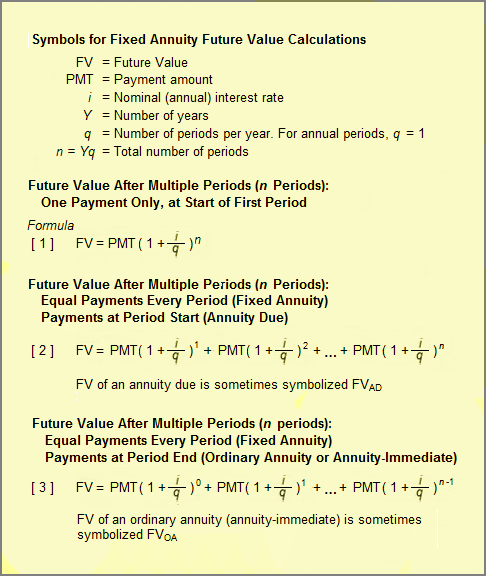Exhibit 1. Formulas and symbols for calculating fixed annuity future values.

#### Example: Future Value, Fixed-Annuity Due (FVAD)

What will be the value of a fixed contract due at the end of its life? Consider an annuity paying the beneficiary  \$100 annually, for five years, with payments coming at the (annual) period start (payment at the period start makes this an annuity due). Use a nominal (annual) interest rate of 8.0%, and re-invest all incoming payments for the life of the contract at the same rate. Using the symbols above,

PMT = \$100
i = 8.0% = 0.08
q = 1
Y = 5
n = Yq = (5)(1) = 5

The resulting cash flow stream is shown graphically in Exhibit 2: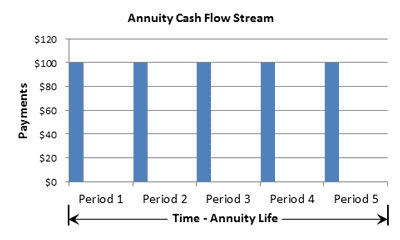Exhibit 2. Cash flow stream for a fixed annuity due (payments appear at the start of each period). When payments arrive at the period start, they earn interest for the period they arrive as well as subsequent periods.

To see interest compounding at work, consider first just the initial \$100 payment. That payment arrives at the start of Period 1 (Year 1), and earns compound interest for 5 periods. The future value of just that payment at annuity end is given by formula  above:

FV = PMT ( 1+ ( i /q ) )n
=  \$100 ( 1+ ( 0.8 / 1 ) )5
=  \$100 (1.08 )5

=  \$100 (1.4693) = \$149,93

For the full payment stream for this annuity due, one payment is compounded five times (as shown), another payment four times before the end of the annuity, another payment is compounded 3 times, and so on. Formula [2} produces the future value (FV or FVAD) of the entire annuity due:

FVAD = \$100 ( 1+ ( 0.8 / 1 ) )1+ \$100 ( 1+ ( 0.8 / 1 ) )+ \$100 ( 1+ ( 0.8 / 1 ) )

+ \$100 ( 1+ ( 0.8 / 1 ) )+ \$100 ( 1+ ( 0.8 / 1 ) )5

= \$100 (1.08)+ \$100 (1.1664)+ \$100 (1.2597) + \$100 (1.3605) + \$100 (1.4693)

= \$633.59

## Fixed-Ordinary-Annuity Future Value FVOA Formulas and Calculations

What will be the value of a fixed ordinary annuity at the end of its life? For the annuity due above, each incoming payment earns interest in the period it arrives and in subsequent periods. However, in the more common ordinary annuity, (or annuity-immediate) payments arrive at the period end and therefore do not begin earning interest until the following period. Formula  produces future value for an ordinary annuity. Formula  subtracts one compounding cycle from each term in formula , by reducing each exponent by 1. Exhibit 3, below, shows the timing of cash flow payments in the ordinary annuity version of this example: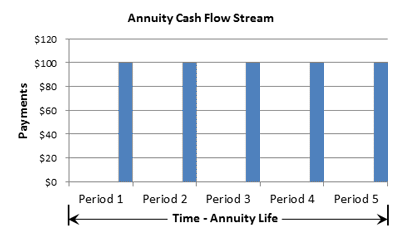Exhibit 3. Cash flow stream for a fixed annuity—ordinary annuity (payments appear at the end of each period). When payments arrive at the period end, they do not earn interest in the next period.

Formula [2} produces the future value (FV or FVOA) of the ordinary annuity:

FVOA = \$100 ( 1+ ( 0.8 / 1 ) )0+ \$100 ( 1+ ( 0.8 / 1 ) )+ \$100 ( 1+ ( 0.8 / 1 ) )

+ \$100 ( 1+ ( 0.8 / 1 ) )+ \$100 ( 1+ ( 0.8 / 1 ) )4

= \$100 (1.0)+ \$100 (1.08)+ \$100 (1.1664) + \$100 (1.2597) + \$100 (1.3605)

= \$586.66

In this case, with 5-period annuities, the difference between the annuity due (FVAD = \$633.59) and the ordinary annuity (FVOA = \$586.66) is relatively large. However, as the number of periods increases (e.g., as with a life annuity or a 30-year mortgage loan), the overall impact of payment timing within the period becomes less important.

## Future Value, Fixed-Ordinary-Annuity FVOA Multiple Periods Per Year

What will be the value of a fixed ordinary annuity at the end of its life, if payments are paid and compounded quarterly? The examples above used annual periods, but consider now another 5-year ordinary annuity with quarterly (3-month) periods (q = 4), with four \$25 payments per year, for a total of 20 periods (n = 20) The same nominal interest rate, 8.0% per year, represents a 2.0% interest rate for each quarterly period (i.e.,  i / q = 0.08/4 = 0.02).  That is, the input variables for formula  are now:

PMT = \$25
i = 8.0% = 0.08
q = 4
Y = 5
n = Yq = (5)(4) = 20

By formula , the future value is now:

FVOA = PMT ( 1+ ( i / q  ) )0+ PMT ( 1+ ( 0.8 / 4 ) )...   + PMT ( 1+ ( .08 / 4 ) )(20 – 1)

= \$25(1.0) + 25 (1.02)1 + \$25 (1.02)2 + \$25 (1.02)3 + \$25 (1.02)4 + ...
...+ \$25 (1.02)17 + \$25 (1.02)18 +\$25 (1.02)19

= \$25 (1.0) + \$25 (1.02)1 + \$25 (1.02)2 + \$25 (1.02)3 + \$25 (1.02)4 + ...
...+ \$25 (1.02)17 + \$25 (1.02)18 + \$25 (1.02)19

= \$25 (1.0000) + \$25 (1.0200) + \$25 (1.0404) + \$25 (1.0612) + \$25 (1.0824) + ...
...+ \$25 (1.4002) + \$25 (1.4282) + \$25 (1.4568)

= \$25 (24.2974)

= \$607.43

(Not all terms appea in intermediate steps above.) The shorter periods in this example compared to the previous example (3 months vs. 1 year) lead to a higher future value(\$607.43 vs. \$586.66). Both examples use the same nominal interest rate (8.0%), but the more frequent payments give the second example a higher effective interest rate (see the encyclopedia entry interest).

## Annuity Notation Example Fixed-Ordinary-Annuity Future Value FVOA formulas and calculations

Insurance companies issue annuities as financial service products. In the insurance industry, annuity metrics belong to the larger body of actuarial calculations. Actuaries present annuity calculations in a special annuity notation (a subset of actuarial notation). Annuity notation examples appear below in Exhibits 4, 5, and 6.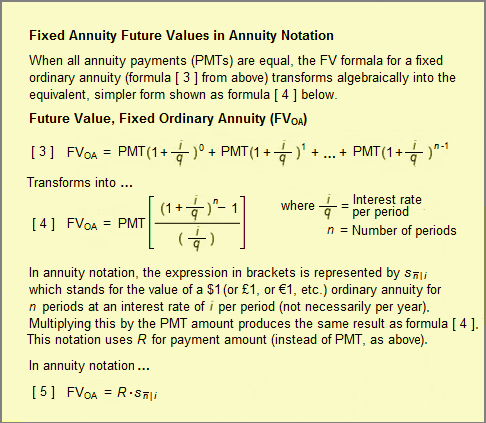Exhibit 4. Symbols and formulas for calculating Fixed annuity future values, in annuity notation."

## Annuity Notation Example Fixed-Ordinary-Annuity Future Value FVOA

How do you find the value of a fixed ordinary annuity at the end of its life, using annuity notation? Future value formula [ 4 ] is applied to the same FVOA example just shown above, for a fixed ordinary annuity, extending five years, with a nominal annual interest rate of 8.0%, with quarterly \$25 payments reinvested for the life of the annuity. That is,

PMT = \$25
i = 8.0% = 0.08
q = 4
Y = 5
n = Yq = (5)(4) = 20
i / q  =  2.0% = 0.02

Using formula [ 4 ]

FVOA = PMT [ ( (1 + ( i / q )n – 1 ) / ( i / q ) ]

= \$25 [ ( ( 1 + 0.02)20 – 1 ) / 0.02  ]

= \$25 [ (0.485947) / 0.02  ]

= \$607.43

This is the same result obtained earlier by computing 20 individual terms in the conventional future value formula [ 3 ].

Annuity notation for the future value of an annuity due (FVAD) is just a simple modification of the future FVOA formula for an ordinary annuity. With an annuity due, payments arrive at the start of each period, thereby earning one more periods interest than a comparable ordinary annuity (where payments arrive at period end). The FVAD annuity notation formula is thus just the FVOA formula multiplied by (1+periodic interest rate), to add the extra compounding period.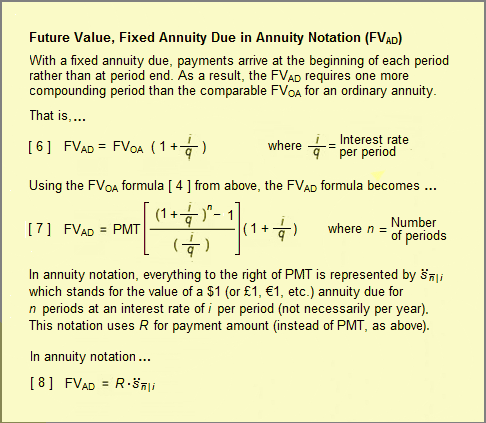Exhibit 5. Formulas and symbols for calculating future values for a fixed annuity due in annuity notation.

## Annuity Notation Example Annuity Notation: Fixed-Annuity-Due Future Value FVAD

How do you find the value of a fixed annuity due (FVAD) at the end of its life, using annuity notation? For the annuity due, payments arrive at the period start rather than the period end, and formula [ 7 ] above captures the extra compounding cycle with the term (1 + i / q ) to the right of the expression in brackets. Consider now the annuity from the previous example, but now cast as an annuity due:  the annuity extends five years, \$25 payments are made quarterly and reinvested at a nominal (annual) interest rate of 8.0% for the remaining life of the annuity. Again, the interest rate per period, i / q, is 8.0% / 4 , or 0.02. That is,

PMT = \$25
i = 8.0% = 0.08
q = 4
Y = 5
n = Yq = (5)(4) = 20
i / q  =  2.0% = 0.02

Using formula [ 7 ]

FVAD = PMT [ ( (1 + ( i / q )n – 1 ) / ( i / q ) ] (1 + i / q)

= \$25 [ ( ( 1 + 0.02)20 – 1 ) / 0.02  ] (1 + 0.08/ 4 )

= \$25 [ (0.485947) / 0.02  ] (1 + 0.02)

= \$619.58

A comparison of the last two examples shows different FV results due to payment timing (payments either at the period start or end). The annuity due has a slight larger future value (FVAD) = \$619.58 compared to the ordinary annuity future value (FVOA=  \$607.43).

## Variable Annuities Future Value Formulas and Calculations

That is the value of a variable annuity at the end of its life? This question is the same future value question appearing above for fixed annuities and—in principle—the same kind of cash flow analysis answers both questions.

Remember, however, that variable annuities have variable payments from period to period because payment for many of these annuities reflects investment performance, or market prices for money market funds, bonds, or other securities. For these annuities, future payments cannot be known with certainty. For such variable annuities, It is better not to think of variable annuity payments and future values as forecasts, but rather as target values that will result if the investment meets its performance goals.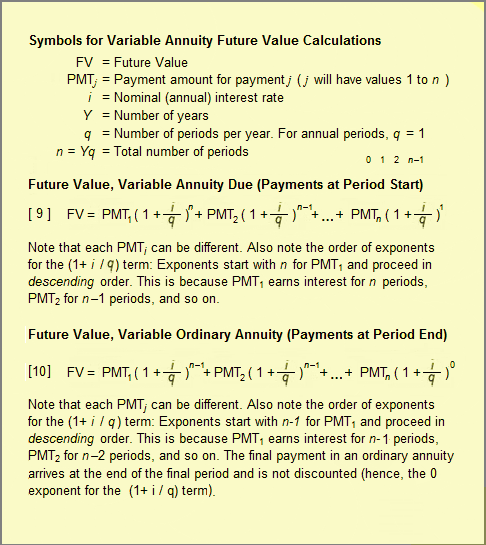Exhibit 6. Formulas and symbols for calculating variable annuity future values.